Disclaimer: This is an example of a student written essay.

Any opinions, findings, conclusions or recommendations expressed in this material are those of the authors and do not necessarily reflect the views of UKEssays.com.

# Symmetry and Group Theory in Relation to Wallpaper Groups

 ✅ Paper Type: Free Essay ✅ Subject: Mathematics ✅ Wordcount: 1528 words ✅ Published: 18th Sep 2017

Mark Anderson

## 1.1 Group Theory

Group Theory was derived from three other areas of mathematics, number theory, the theory of algebraic equations and geometry. The first prominent mathematicians credited with studying group theory were A-L.Cauchy, E.Galois and J-L.Lagrange. Although Lagrange’s work with groups is probably the earliest research into groups, in the 18th century, his work was rather isolated and the mid-19th century works of Cauchy and Galois are often considered to be the origin of the study of group theory.

In his 1770 paper, Lagrange was the first mathematician to study permutations. His objective for the study was to discover why cubic and quartic equations could be solved using the theory of algebra. During his work, while evident permutation group theory is being used in his work, the permutations are never composed and he never discusses groups themselves.

Cauchy published his first paper on the topic of permutations in 1815, however, it was not until his work in 1844 that permutations were considered a subject in its own right by introducing many of the key aspects of permutation groups including the notation of positive and negative powers of groups, identifying the power 0 being the identity, the cycle and permutation notation of a group and the order of a permutation. He also proved the conjugacy of permutations if the permutations have the same cycle structure and Cauchy’s theorem “If a prime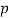divides the order of a group, that group has a subgroup of order“.

Galois’ had papers published posthumously in 1846 by Liouville after Liouville saw a connection between Galois work and the permutation work of Cauchy from 1844. This work showed that Galois understood the relationship between the structure of a group of permutations related to the equation and the algebraic solution of an equation. To fully show this he created the notion of a normal subgroup. This was the first time the term “group” had been used in a technical sense.

If you need assistance with writing your essay, our professional essay writing service is here to help!

## 1.2 Wallpaper Groups

The origins of the study of wallpaper groups began as the study of crystallography which was to determine the structure of crystalline solids at the atomic level. It was from this study that many of the proofs for symmetry were discovered and set the foundations for more advanced symmetry groups such as space group which led to the proof of wallpaper groups.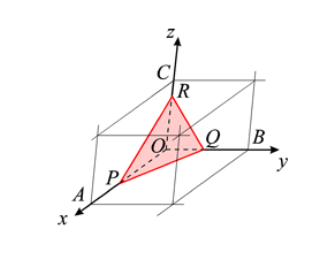In 1830, J.F.C Hessel discovered the maximum unique combinations of reflections and rotations of a crystal around a fixed point such that the image created is symmetrical to be 32. He proves this using the law of indices which states “that the intercepts, OP, OQ, OR, of the natural faces of a crystal form with the unit-cell axes a, b, c are inversely proportional to prime integers, h, k, l.” (IUCR, 2016). This is illustrated in the image below.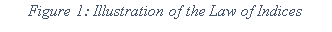All 32 combinations with crystallographic symmetry were then found geometrically in 1835 by M.L Frankenheim.

Using the theory of crystal classes discovered by Hessel, A. Bravais systemized the theory and classified the 14 spacial lattices, which we now know as Bravais Lattices. These lattices are defined as infinite arrays of discrete points in a 3-Dimensional plane created by a set of operations described by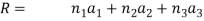Using both Hessel’s and Bravais’ work E. Fedorov and A.M Schönflies proved the existence of the 230 space groups in 1891. These space groups are Bravais lattices that have been reflected or rotated in any of the 32 unique point groups discovered by Hessel. These space groups within a 2-dimensional plane are the 17 wallpaper groups, which although known for centuries was only proved after the proof of space groups was already completed.

A group is defined as a non-empty set under a binary operation, i.e. addition, multiplication etc. In order to be classified as a group, it must maintain four conditions: closure, associativity, identity and inverse.

Let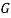be a group with a binary operation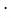Closure: For every element belonging to the group, the result of every two elements under the binary operation on the group is equal to another element of the group. i.e.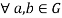then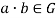.

Associativity: If three elements belong to the groupthen the order the operation is performed on the three elements will not affect the outcome. i.e. If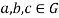the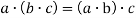.

Identity: There exists an element in the group such that when the binary operation is applied to it and any other element in the group, the outcome is equal to the other element. i.e.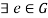such that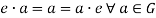.

Inverse: For every element in the groupthere is another element insuch that when the two elements are under the operation the outcome is equal to the identity. i.e.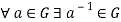such that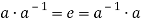A simple example of a group is the group of integers under the operation of addition (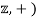. This can be proved by showing the group satisfies the four axioms as stated above. Any integer added to any other is another integer, so the group is closed. Addition is associative, the identity of the group is 0 as any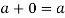, and the inverse of any integer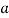is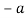as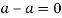.

A basic symmetry group to understand how symmetry is related to group theory is the symmetries of the rectangle. This group contains the linear transformations that leave the rectangles origin in place i.e. rotations and reflections. This shows there are 4 symmetries of the rectangle as shown in Figure 2.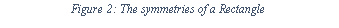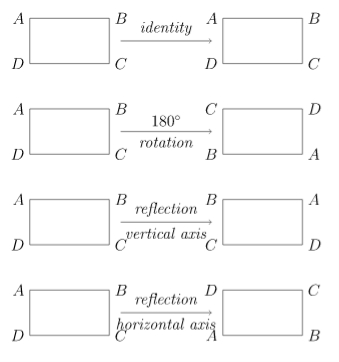This can be displayed in multiple ways including Cayley tables, matrices and as permutations

References

International Union of Crystallography, 2016. Law of Rational Indices. [Online]
Available at: http://reference.iucr.org/dictionary/Law_of_rational_indices
[Accessed 26 February 2017].

Kleiner, I., 2004. The Evolution of Group Theory: A Brief Survey. [Online]
[Accessed 24 February 2017].

O’Connor, J. J. & Robertson, E. F., 1996. The Development of Group Theory. [Online]
Available at: http://www-groups.dcs.st-and.ac.uk/history/HistTopics/Development_group_theory.html
[Accessed 24 February 2017].

View all

## DMCA / Removal Request

If you are the original writer of this essay and no longer wish to have your work published on UKEssays.com then please:

Related Services

Prices from

S\$203

Approximate costs for:

• 1000 words
• 7 day deliveryHumanity University

Dedicated to your worth and value as a human being!

Related Lectures